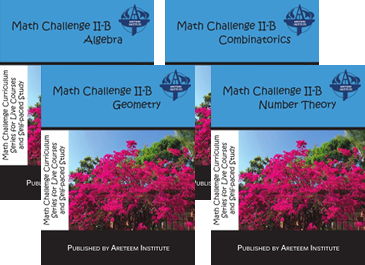# Math Challenge II-B Self-paced

### Course Description

In Math Challenge II-B, students learn and practice in areas such as algebra and geometry at the high school level, as well as advanced number theory and combinatorics. Topics include polynomials, inequalities, special algebraic techniques, trigonometry, triangles and polygons, collinearity and concurrency, vectors and coordinates, numbers and divisibility, modular arithmetic, residue classes, advanced counting strategies, binomial coefficients, pigeonhole principle, sequence and series, and various other topics and problem solving techniques involved in math contests such as the American Mathematics Competition (AMC) 10/12, ARML, and also beginning AIME.

### Course Textbooks

Each term requires the course textbook:The textbook is available in print (for \$25 each) on our online bookstore (click here) or eBook (rental starting at \$10 each) on redshelf.com (click here). More information about the textbooks, including answer keys, is available by clicking here.

### Course Terms

Math Challenge II-B is offered in four terms:

• Algebra
• Geometry
• Combinatorics
• Number Theory

We recommend that students take all four terms, but the terms do not build on previous terms, so they do not need to be taken in order and students can take single terms if they want to focus on specific topics. For more information about the topics covered each term, please click here.

### Registration and Tuition

Click on any of the courses below to register! You will be prompted to login or create a new account if you have not already done so. Registration options include:

• Single Term, $380 with access for 4 months (120 days) • Four Course Bundle,$1368 including all four terms with access for 1 year (365 days)

Each MC II-B self-paced course includes:

• 10 Video Lectures covering selected Example Problems
• 9 Quizzes which test knowledge using the quick response questions and provide answers and outlines of solutions to all the problem solving questions.
• A final quiz consisting of problems done throughout the course, which can be used as a final assessment once all the handouts are completed.

### MC II-B Self-Paced Year BundleStudents enrolled in this packet have access to all 4 terms of Math Challenge II-B. Students have one year to complete the 4 terms.

Each term will have its own textbook available for rent (as an eBook) or purchase (as an eBook or physical copy).

### MC II-B Algebra Self-PacedThe focus of this term of Math Challenge II-B is algebra. Students have 4 months to complete the course.

The required textbook for this course (MC II-B Algebra) is available as an eBook (click here) and as a physical copy (click here).

### MC II-B Geometry Self-PacedThe focus of this term of Math Challenge II-B is geometry. Students have 4 months to complete the course.

The required textbook for this course (MC II-B Geometry) is available as an eBook (click here) and as a physical copy (click here).

### MC II-B Combinatorics Self-PacedThe focus of this term of Math Challenge II-B is combinatorics. Students have 4 months to complete the course.

The required textbook for this course (MC II-B Combinatorics) is available as an eBook (click here) and as a physical copy (click here).

### MC II-B Number Theory Self-PacedThe focus of this term of Math Challenge II-B is number theory. Students have 4 months to complete the course.

The required textbook for this course (MC II-B Number Theory) is available as an eBook (click here) and as a physical copy (click here).